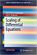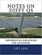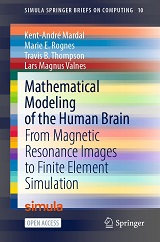Processing ......FreeComputerBooks.com Links to Free Computer, Mathematics, Technical Books all over the World

A Friendly Introduction to Differential Equations
Top Free C Programming Books 🌠 - 100% Free or Open Source!
• Title: A Friendly Introduction to Differential Equations
• Author(s) Mohammed K A Kaabar
• Publisher: CreateSpace; 1 edition (January 5, 2015); eBook (Creative Commons Licensed)
• Paperback: 164 pages
• eBook: HTML and PDF (165 pages)
• Language: English
• ISBN-10: 1506004539
• ISBN-13: 978-1506004532Book Description

In this book, there are five chapters: The Laplace Transform, Systems of Homogenous Linear Differential Equations (HLDE), Methods of First and Higher Orders Differential Equations, Extended Methods of First and Higher Orders Differential Equations, and Applications of Differential Equations.

In addition, there are exercises at the end of each chapter above to let students practice additional sets of problems other than examples, and they can also check their solutions to some of these exercises by looking at Answers to Odd-Numbered Exercises section at the end of this book.

This book is a very useful for college students who studied Calculus II, and other students who want to review some concepts of differential equations before studying courses such as partial differential equations, applied mathematics, and electric circuits II.

• Mohammed K A Kaabar has a Bachelor of Science in Theoretical Mathematics from Washington State University, Pullman, WA. He is a graduate student in Applied Mathematics at Washington State University, Pullman, WA, and he is a math tutor at the Math Learning Center (MLC) at Washington State University, Pullman.
Reviews, Ratings, and Recommendations: Related Book Categories: Read and Download Links:Similar Books:
•Scaling of Differential Equationsn (Hans Langtangen, et al.)

Scaling is a classical topic in applied mathematics, but here strongly connected to numerical simulations. The book contains a wide range of examples, of differing complexity, from many different scientific fields.

•Notes on Diffy Qs: Differential Equations for Engineers (Jiri Lebl)

An introductory course on differential equations aimed at engineers. The book covers first order ODEs, higher order linear ODEs, systems of ODEs, Fourier series and PDEs, eigenvalue problems, the Laplace transform, and power series methods.

•Finite Difference Computing with PDEs: A Software Approach

This easy-to-read book introduces the basics of solving partial differential equations by means of finite difference methods. Unlike many of the traditional academic works on the topic, this book was written for practitioners.

•Solving PDEs in Python: The FEniCS Tutorial I (H. Langtangen)

This book offers a concise and gentle introduction to finite element programming in Python based on the popular FEniCS software library. Using a series of examples, it guides readers through the essential steps to quickly solving a PDE in FEniCS.

•Elementary Differential Equations (William F. Trench)

This text has been written in clear and accurate language that students can read and comprehend. The author has minimized the number of explicitly state theorems and definitions, in favor of dealing with concepts in a more conversational manner.

•Elementary Differential Equations with Boundary Value Problems

Written in a clear and accurate language that students can understand, this book minimizes the number of explicitly stated theorems and definitions, includes a thorough treatment of boundary-value problems and partial differential equations.

•Stochastic Differential Equations: Models and Numerics

The goal of this book is to give useful understanding for solving problems formulated by stochastic differential equations models in science, engineering and mathematical finance. Typically, these problems require numerical methods to obtain a solution.

•Mathematical Modeling of the Human Brain

The book bridges common tools in medical imaging and neuroscience with the numerical solution of brain modelling PDEs, covers the basics of magnetic resonance imaging and quickly proceed to generating first FEniCS brain meshes from T1-weighted images.

•Linear Partial Differential Equations and Fourier Theory

This highly visual introductory textbook presents an in-depth treatment suitable for undergraduates in mathematics and physics, gradually introducing abstraction while always keeping the link to physical motivation.

Book Categories
 :All CategoriesTop Free BooksRecent BooksMiscellaneous BooksComputer EngineeringComputer LanguagesComputer ScienceData Science/DatabasesJava and Java EE (J2EE)Linux and UnixMathematicsMicrosoft and .NETMobile ComputingNetworking and CommunicationsSoftware EngineeringSpecial TopicsWeb Programming
Other Categories